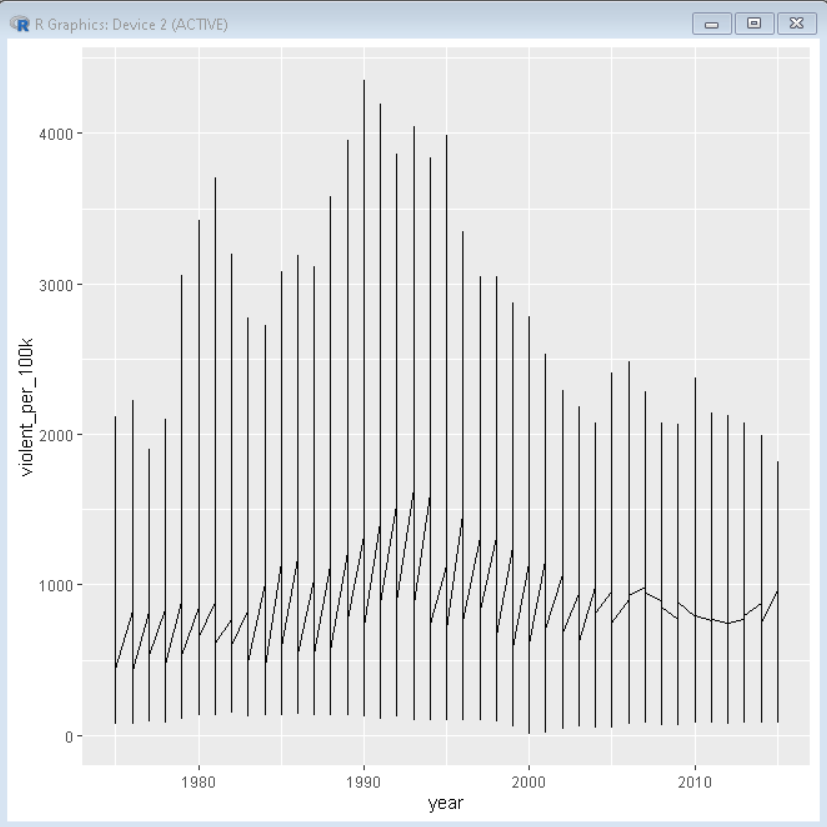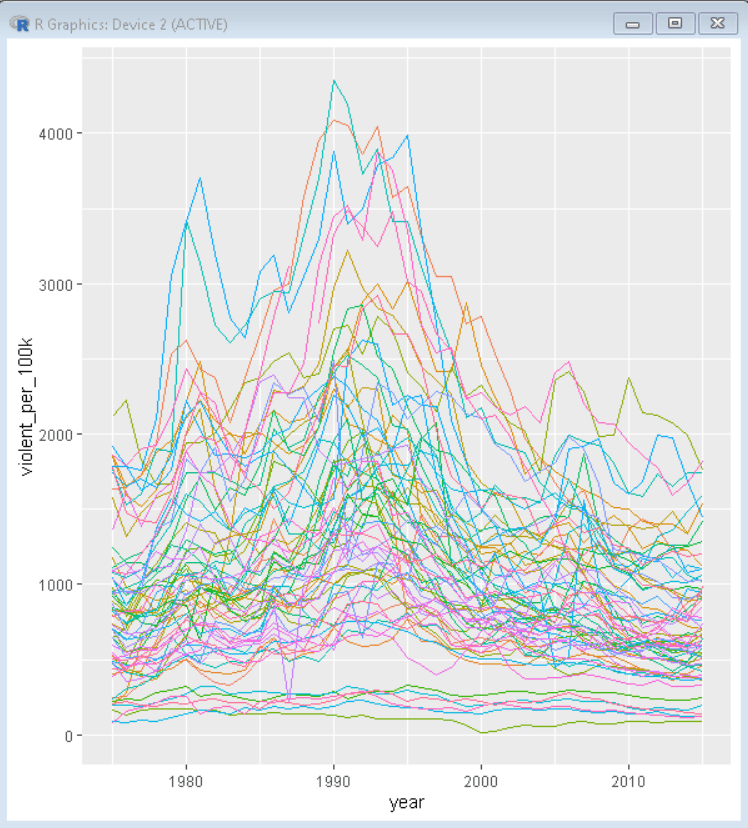Open in App
Not now

# Multiple Line Plots or Time Series Plots with ggplot2 in R

• Last Updated : 24 Oct, 2021

In this article, we will discuss how to plot Multiple Line Plots or Time Series Plots with the ggplot2 package in the R Programming Language. We can create a line plot using the geom_line() function of the ggplot2 package.

Syntax:

ggplot( df, aes( x, y ) ) + geom_line()

where,

• df: determines the dataframe used
• x and y: determines the axis variables

Example:

Here, is a basic line plot made using the geom_line() function of the ggplot2 package. Here, the dataset used is the city crime dataset from 1975 to 2015 which can be downloaded from ucr_crime.

## R

 `# load package tidyverse``library``(tidyverse) `` ` `# read sample data from given csv file``sample_data <- ``read_csv``(``"ucr_crime_1975_2015.csv"``)`` ` `# plot a basic line plot using ggplot() function``# color parameter is used to colot the plot according ``# to department_name``ggplot``(sample_data, ``aes``(x=year, violent_per_100k)) +`` ` `# geom_line function is used to plot line plot``geom_line``()`

Output:Here, the resulting plot doesn’t look like multiple time series. It is because for a multiple time series in the above example we just used two variables and those two are needed for a single time series plot. To get a multiple time series plot we need one more differentiating variable. So we will use the color parameters to group and color the line plot according to another variable of differentiating caliber.

Syntax:

ggplot( df, aes( x, y, color ) ) + geom_line()

where,

• df: determines the data frame used
• x and y: determines the axis variable
• color: determines the variable according to which plot is grouped and colored

Example:

Here, is a basic line plot made using the geom_line() function of the ggplot2 package. Here we have grouped and colored the plot according to department_name. This creates the necessary three differentiating variables for multiple time series.

## R

 `# load package tidyverse``library``(tidyverse) `` ` `# read sample data from given csv file``sample_data <- ``read_csv``(``"ucr_crime_1975_2015.csv"``)`` ` `# plot a basic line plot using ggplot() function``# color parameter is used to colot the plot according``# to department_name``ggplot``(sample_data, ``aes``(x=year, violent_per_100k, color=department_name)) +`` ` `# geom_line function is used to plot line plot``geom_line``()+`` ` `# theme with legend.position as none removes the ``# legend form plot``theme``(legend.position = ``"none"``)`

Output:My Personal Notes arrow_drop_up